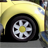Announcements
Applications are open for the 2024 Qlik Luminary Program. Apply by December 15 here.
cancel
Showing results for
Did you mean:Creator

## Alt() Function with Applied Filter

Hello All,

I am trying to create a measure in Qlik Sense that is a conversion rate between two stages within a process, but I want to be able to change the stages based on a filter (or another way if it is easier).

My stages are as follows:

[Prospects]

[Application Started]

[Application Completed]

[Processing]

[Funded]

I want to be able to see the conversion rate between any two of these stages by applying a filter. For example, the conversion rate between [Prospects] and [Funded] would be Count([Funded]) / Count([Prospects]), and the conversion rate between [Application Started] and [Application Completed] would be Count([Application Completed]) / Count([Application Started]). Easy enough.

The problem is that I don't want 10 KPI boxes to be able to show all possible conversion rates. I want one KPI box that will display a different conversion rate depending on what I select (likely in a filter box). For example, if I filter the Stage to [Application Started] and [Funded], the KPI box would automatically display the conversion rate between those two fields.

I tried to use the Alt() function, but I'm not sure if I'm going in the right direction. I tried this:

Alt(Sum([Funded]),Sum([Processing]),Sum([Application Completed]),Sum([Application Started]),Sum([Prospects]))

/

Alt(Sum([Prospects]),Sum([Application Started]),Sum([Application Completed]),Sum([Processing]),Sum([Funded]))

I figured 1) that if there were no filter applied, the KPI box would display the conversion from [Prospects] to [Funded] (first to last), and 2) that if a filter were applied, it would display the conversion rate between the first and last stages selected. The first is true and works just fine; however, if a filter that excludes either [Prospects] or [Funded] is applied, the KPI box displays nothing.

Please let me know if there is a way to fix this.

Thanks!

Aaron

1 Solution

Accepted SolutionsSpecialist III

Hi Aaron,

i would prefer to create a InLine Table:

[

Stage,StageNo

[Prospects],1

[Application Started],2

[Application Completed],3

[Processing],4

[Funded],5

]

;

Then write the expression something like this.

=if(GetSelectedCount(Stage)=0 or

if(GetSelectedCount(Stage)>1

,Count({<StageNo={"=Min(StageNo"}>}Stage)/Count({<StageNo={"=Max(StageNo"}>}Stage)

if(GetSelectedCount(Stage)=1,Count({<StageNo={"Only(StageNo)"}>}Stage)/Count({1}Funded)))

Thanks,

Mukram

3 RepliesSpecialist

Hi Aaron,

Have you tried just using the GetCurrentSelections(), GetFieldSelections() , GetSelectedCount(), etc and then in the KPI apply the appropriate formula based on the selection?

Cheers,

LuisCreator
Author

Luis,

I've read up on those functions and I haven't seen how they would be able to help with this situation. Could you be a little more specific as to how I could convert one of these functions to a sum of the desired field?

Thank you for the help.

AaronSpecialist III

Hi Aaron,

i would prefer to create a InLine Table:

[

Stage,StageNo

[Prospects],1

[Application Started],2

[Application Completed],3

[Processing],4

[Funded],5

]

;

Then write the expression something like this.

=if(GetSelectedCount(Stage)=0 or

if(GetSelectedCount(Stage)>1

,Count({<StageNo={"=Min(StageNo"}>}Stage)/Count({<StageNo={"=Max(StageNo"}>}Stage)

if(GetSelectedCount(Stage)=1,Count({<StageNo={"Only(StageNo)"}>}Stage)/Count({1}Funded)))

Thanks,

MukramTags
Community Browser